Test: Area Of Parallelograms

# Test: Area Of Parallelograms

Test Description

## 10 Questions MCQ Test NCERT Mathematics for CAT Preparation | Test: Area Of Parallelograms

Test: Area Of Parallelograms for Class 9 2022 is part of NCERT Mathematics for CAT Preparation preparation. The Test: Area Of Parallelograms questions and answers have been prepared according to the Class 9 exam syllabus.The Test: Area Of Parallelograms MCQs are made for Class 9 2022 Exam. Find important definitions, questions, notes, meanings, examples, exercises, MCQs and online tests for Test: Area Of Parallelograms below.
Solutions of Test: Area Of Parallelograms questions in English are available as part of our NCERT Mathematics for CAT Preparation for Class 9 & Test: Area Of Parallelograms solutions in Hindi for NCERT Mathematics for CAT Preparation course. Download more important topics, notes, lectures and mock test series for Class 9 Exam by signing up for free. Attempt Test: Area Of Parallelograms | 10 questions in 10 minutes | Mock test for Class 9 preparation | Free important questions MCQ to study NCERT Mathematics for CAT Preparation for Class 9 Exam | Download free PDF with solutions
 1 Crore+ students have signed up on EduRev. Have you?
Test: Area Of Parallelograms - Question 1

### Two parallelograms are on equal bases and between the same parallels. The ratio of their areas is:

Detailed Solution for Test: Area Of Parallelograms - Question 1

Two parallelograms are on equal bases and between the same parallels.
i.e., their corresponding heights must be the same.
Thus, areas of both parallelograms are equal. Hence, the ratio of their areas is 1:1.

Test: Area Of Parallelograms - Question 2

###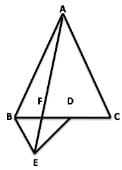In the figure, ABC and BDE are two equilateral triangles such that D is the midpoint of BC. If AE intersects BC at F, then

Detailed Solution for Test: Area Of Parallelograms - Question 2

Let the altitude of the triangle ABC is h

Then altitude of the triangle BED = h/2

Now, ar(Δ FED) = (1/2)*FD*(h/2) = (FD  * h)/2

and ar(Δ AFC) = (1/2)*FC*h

= (1/2)*(FD + DC)*h

= (1/2)*(FD + BD)*h

= (1/2)*(FD + BF +  FD)*h

= (1/2)*(2FD + BF)*h

= (1/2)*(2FD + 2FD)*h

= 2FD*H

⇒  ar(Δ AFC)/8 = 2FD*H/8

⇒  ar(Δ AFC)/8 = 2FD*H/4

⇒  ar(Δ AFC)/8 = ar(Δ FED)

Test: Area Of Parallelograms - Question 3

### In parallelogram ABCD, AL ⊥ CD and CM ⊥ AD. If AL=20cm, CD = 12 cm, CM=8cm, then perimeter of the parallelogram is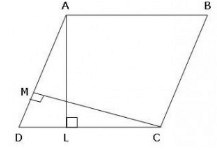Detailed Solution for Test: Area Of Parallelograms - Question 3

Given that ABCD is a IIgm

AL = 20 cm

CD = 12 cm

CM = 8 cm

Area of IIgm ABCD will be = BASE * ALTITUDE

= AL * CD

= 20 * 12= 240 cm2

Again taking base AD and Altitude MC

than Ar IIgm ABCD = B * h

or  AD = 240/8 = 30 cm

now ,

CD = AB = 12 cm (opp. sides of a IIgm)

AD = BC = 30 cm(opp. sides of a IIgm)

now ,

Perimeter of IIgm ABCD =

2a + 2b

or 2(a+b)

2 (30+12)

2(42)

or, 42*2 = 84 cm

Test: Area Of Parallelograms - Question 4

PQRS is a trapezium in which PQ = 7 cm, PS = QR = 5 cm, RS = x cm and distance between PQ and RS is 4 cm. The area of trapezium is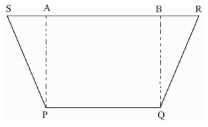Test: Area Of Parallelograms - Question 5

A triangle and a rhombus are on the same base and between the same parallels. Then the ratio of area of triangle to that rhombus is:

Detailed Solution for Test: Area Of Parallelograms - Question 5

If a triangle and a rhombus are on the same base and between the same parallels then the ratio of the areas of the triangle and rhombus is 1 : 2

The base of triangle and rhombus are equal. The rhombus have equal sides, and  one side is the base of triangle.

The are of triangle must be half of the are of rhombus.

Test: Area Of Parallelograms - Question 6

ABCD is a parallelogram of area 100 cm2. AE is drawn perpendicular to BC and AF is drawn perpendicular to DC. If AE = 8 cm and AF is equal to 6.25 cm, then AB is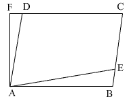Detailed Solution for Test: Area Of Parallelograms - Question 6

Area = B x H
100 = DC x AF
100 = DC x 6.25
DC = 100/6.25
DC = 16cm
AB = DC( in a parallelogram opposite sides are equal )

So AB = 16cm

Test: Area Of Parallelograms - Question 7

Parallelogram ABCD and rectangle ABEF are on the same base AB. If AB = 14 cm, BC = 12 cm, then the possible value for the perimeter of ABEF is

Detailed Solution for Test: Area Of Parallelograms - Question 7

IIgm ABCD and rectangle ABEF are between the same parallels AB and CF.
AB = EF (For rectangle) and AB = CD (For parallelogram)
? CD = EF ? AB + CD = AB + EF ... (1)
Of all the line segments that can be drawn to a given line from a point not lying on it, the perpendicular line segment is the shortest.
similarly we write , BE < BC ? AF + BE < AD + BC ... (2)
From equations (1) and (2), we get
AB + EF + AF + BE < AD + BC + AB + CD
Perimeter of rectangle ABEF < Perimeter of parallelogram ABCD

Now,  Perimeter of parallelogram ABCD = AB + BC + CD + DA = 14 + 12 + 14 + 12 = 52

Thus, Perimeter of rectangle ABEF < 52 cm

Test: Area Of Parallelograms - Question 8

ABCD is a parallelogram, Area(DAB) =

Detailed Solution for Test: Area Of Parallelograms - Question 8

ABCD is a parallelogram
In a Triangle ABC and ADC
AB=CD(opposite lines of a parallelogram)
AC=AC(common)
Triangle ABC and ADC ( SSS Cong. rule)
Hence proved.

Test: Area Of Parallelograms - Question 9

In the following figure, if ED = 10 cm, BG = 8 cm and BE = CD = 9 cm, then find the area of triangle CDF.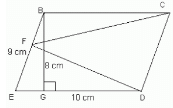Detailed Solution for Test: Area Of Parallelograms - Question 9

If a triangle and a parallelogram are on the same base and between the same parallels, then the area of the triangle is equal to half the area of the parallelogram.

Here, the area of the parallelogram = base x height = 10 x 8 = 80 cm2

Therefore, the area of the triangle = 1/2 x area of parallelogram

= 1/2 x 80 = 40 cm2

Test: Area Of Parallelograms - Question 10

Find the area of quadrilateral ABCD, if AB || CD and AB = CD is as shown in figure :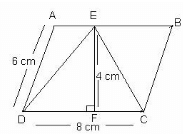Detailed Solution for Test: Area Of Parallelograms - Question 10

This is a condition of parallelogram

Area = EF×DC =4  cm × 8 cm = 32 sq. cm

## NCERT Mathematics for CAT Preparation

276 docs|149 tests
 Use Code STAYHOME200 and get INR 200 additional OFF Use Coupon Code
Information about Test: Area Of Parallelograms Page
In this test you can find the Exam questions for Test: Area Of Parallelograms solved & explained in the simplest way possible. Besides giving Questions and answers for Test: Area Of Parallelograms, EduRev gives you an ample number of Online tests for practice

## NCERT Mathematics for CAT Preparation

276 docs|149 tests